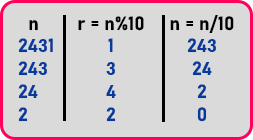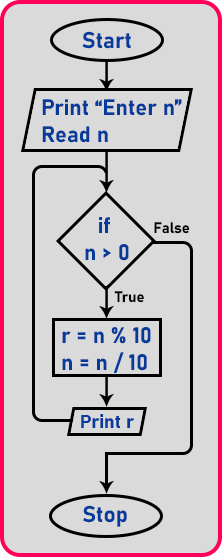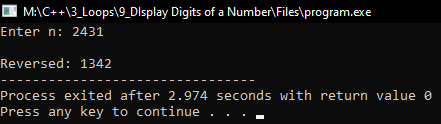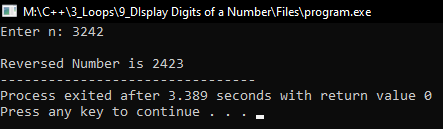# Display Digits of a Number using Loop in C++

## Display Digits of a Number using Loop in C++

In this article, I am going to discuss How to Display Digits of a Number using Loop in C++ with Examples. Please read our previous articles, where we discussed Prime Number using Loop in C++ with Examples. Here, you will learn about how to display the digits of a number from the reverse. If any number is given then we will display the digits.

##### Display Digits of a Number:

Let us take the number ‘n = 2431’, and we want to display it as ‘1342’. So, to print the number in reverse, we want each digit separately. Now let us show you the procedure to get the digits of a number. The Procedure is as follows:

###### Step 1:

So, how we can get the last digit? In the number 2431, the last digit is 1. To get the last digit of a number, we will use mod operator %, let see,
2431 % 10 = 1
Here we have used mod operator with 10, the mod will give the remainder, and also, we want the last digit so here we have used mod with 10. Now how to get 3? To get the digit 3 we have to make 3 as the last digit of the number. How to make 3 the last digit? We have to remove 1. To do that, we will divide the number by 10 and then we got the quotient as the result, let see,
2431 / 10 = 243
Here we have performed integer division, decimal part will not be considered here. Now the number has been reduced to 243 from 2431. Now 3 is the last digit.

###### Step 2:

Now the number is modified to 243, so for getting the digit we will mod this with 10,
243 % 10 = 3
We got 3 here, now next we want to make 4 as the last digit, so for making the previous digit as the last digit, we will divide the number with 10,
243 / 10 = 24
Here 4 is the last digit.

###### Step 3:

Now the number is 24. Here also we will do same as previous steps. For getting the digit,
24 % 10 = 4
To make 2 the last digit,
24 / 10 = 2

###### Step 4:

The number is 2. For getting the digit,
2 % 10 = 2
As we do with all the digits, now 2 is already the last digit. We have to store a particular digit that we are getting from the number in a variable. Let’s say the variable is r. Below is the table of the above steps,So, this was the procedure for extracting the digits from a number. We will implement this procedure into our program but before that let’s see the flowchart for reversing the digit of a number.

##### Display Digits of a Number Flowchart:Let us explain this flowchart. First, we will take a number from the user that is ‘n’. Next, we will check the condition ‘if (n > 0)’, if yes then process 2 steps

1. r = n % 10 (To get the last digit of the number).
2. n = n / 10 (To make the previous digit as the last digit of the number).

And then print the value of ‘r’ on the screen. After printing continues to the same steps until the condition becomes false. If the condition ‘if (n > 0)’ is false then exit the loop. Now we have seen the procedure as well as the flowchart, now let us see the implementation of this procedure in the program.

##### Program to print digits in reverse order of a number in C++:
```#include <iostream>
using namespace std;
int main()
{
int n, r;
cout << "Enter n: ";
cin >> n;
cout << endl;
cout << "Reversed: ";
while (n > 0)
{
r = n % 10;
n = n / 10;
cout << r;
}
return 0;
}
```
###### Output:##### Program to print the reverse of a number using loop in C++:
```#include <iostream>
using namespace std;
int main()
{
int n, r, rev = 0, m;
cout << "Enter n: ";
cin >> n;
cout << endl;
m = n;
while (n > 0)
{
r = n % 10;
n = n / 10;
rev = rev * 10 + r;
}
cout << "Reversed Number is " << rev;
return 0;
}
```
###### Output:1.